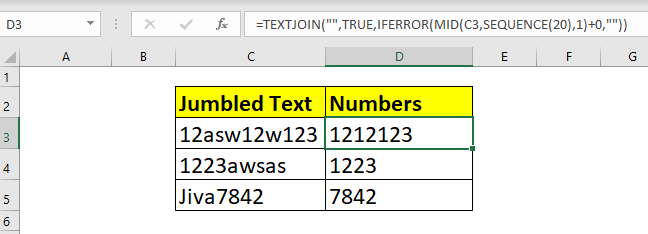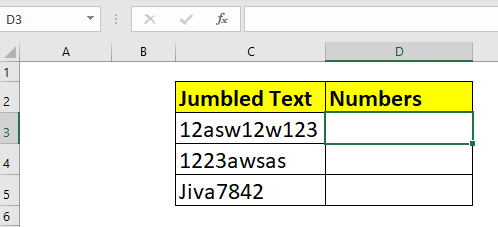# How to Remove Non Numeric Characters From Cells in ExcelWe have learned how to strip numeric values from a cell in excel 2016 and older. The formulas we used were a little bit complex but now Excel 2019 and 365 are in the game.

Excel 2019 and 365 introduce some new functions that ease the task of removing non numeric characters and retrieve only numeric values in a new cell. We will use formulas that can help us in doing so, more conveniently.

Generic Formula

 =TEXTJOIN("",TRUE,IFERROR(MID(jumbled_text,SEQUENCE(NumChars),1)+0,""))

Jumbled_text: This is the source text from which you want to extract all numeric values.

NumChars: This is the total number of characters you want to process. The jumbled_text should not have more characters than this number (chars and numeric combined).

Let’s see an example to make things clear.

## Example: Remove Non Numeric Characters and Extract all NumbersSo here we have some jumbled text. This text contains some numbers and some non numeric characters. I need to get rid of non numeric characters and get numeric values only in the D column.

I don't expect the total number of characters in jumbled text to be more than 20. So the value of NumChars is 20 here. You can increase this number if you need to.

Apply the above generic formula here to strip out the non numeric characters.

 =TEXTJOIN("",TRUE,IFERROR(MID(C3,SEQUENCE(20),1)+0,""))

And when you hit the enter button. You get all the non numeric characters removed. Drag down this formula to remove characters from string from all the cells in column C3.How does it work?

First let's see how this formula is solved step by step.

 1-> TEXTJOIN("",TRUE,IFERROR(MID(C3,SEQUENCE(20),1)+0,"")) 2-> TEXTJOIN("",TRUE,IFERROR(MID("12asw12w123",{1;2;3;4;5;6;7;8;9;10;11;12;13;14;15;16;17;18;19;20},1)+0,"")) 3-> TEXTJOIN("",TRUE,IFERROR({"1";"2";"a";"s";"w";"1";"2";"w";"1";"2";"3";"";"";"";"";"";"";"";"";""}+0,"")) 4-> TEXTJOIN("",TRUE,IFERROR({1;2;#VALUE!;#VALUE!;#VALUE!;1;2;#VALUE!;1;2;3;#VALUE!;#VALUE!;...;#VALUE!}+0,"")) 5-> TEXTJOIN("",TRUE,{1;2;"";"";"";1;2;"";1;2;3;"";"";"";"";"";"";"";"";""}) 6-> "1212123"

As you can see, the formula starts solving from the inside. At first the SEQUENCE function is solved. Since we have passed 20. It returns an array of numbers starting from 1 to 20.

This array is served to the MID Function as the starting number. The mid function goes to each index in string and splits each character. You can see that in step 3.

Next we add 0 to each character. In excel if you try to add number to non numeric characters, it results into #VALUE! Error. So we get an array of numbers and #VALUE! Errors. The non numeric characters are gone now.

Next IFERROR function replaces all #VALUE errors with "" (blank). Now we are left with numeric values and spaces.

Finally, this array is served to TEXTJOIN Function. The TEXTJOIN function concatenates them and we get the a string that only contains numbers in it.

Enhancing the Formula

The above formula used a hard coded number for processing the number of characters (we took twenty). But you may want it to be dynamic. In that case, you guessed it right, we will use the LEN function. It will take an exact number of characters for processing. So the formula will be.

 =TEXTJOIN("",TRUE,IFERROR(MID(jumbled_text,SEQUENCE(LEN(jumbled_text)),1)+0,""))

Here, the LEN function will automatically detect the exact number characters in the alphanumeric string. This will relieve the burden of determining the maximum number of characters.

Alternative of SEQUENCE function

If you do not want to use the SEQUENCE function then you can use a combination of ROW and INDIRECT function  for generating sequential numbers.

 =TEXTJOIN("",TRUE,IFERROR(MID(jumbled_text,ROW(INDIRECT("1:"&LEN(jumbled_text))),1)+0,""))

The INDIRECT will convert the text ("1:20") into actual range and then the ROW function will list all row numbers from 1 to 20. (20 is just for example. It can be any number).

So yeah guys, this is how you can rip off the non numeric characters from an alphanumeric string in excel. I hope I was explanatory enough and this article helped you. If you have any questions regarding this topic or any other excel/VBA topic. Till then keep Excelling.

Related Articles:

Split Numbers and Text from String in Excel 2016 and Older: When we didn't have TEXTJOIN function we used LEFT and RIGHT functions with some other functions to split numeric and nonnumeric characters from a string.

Extract Text From A String In Excel Using Excel's LEFT And RIGHT Function: To remove text in excel from string we can use excel's LEFT and RIGHT function. These functions help us chop strings dynamically.

Remove leading and trailing spaces from text in Excel: Leading and trailing spaces are hard to recognize visually and can mess up your data. Stripping these characters from the string is a basic and most important task in data cleaning. Here's how you can do it easily in Excel.

Remove Characters From Right: To remove characters from the right of a string in Excel, we use the LEFT function. Yes, the LEFT function. The LEFT function retains the given number of characters from LEFT and removes everything from its right.

Remove unwanted characters in Excel: To remove unwanted characters from a string in Excel, we use the SUBSTITUTE function. The SUBSTITUTE function replaces the given characters with another given character and produces a new altered string.

How to Remove Text in Excel Starting From a Position in Excel: To remove text from a starting position in a string, we use the REPLACE function of Excel. This function help us determine the starting position and number of characters to strip.

Popular Articles:

50 Excel Shortcuts to Increase Your Productivity | Get faster at your task. These 50 shortcuts will make you work even faster on Excel.

How to use Excel VLOOKUP Function| This is one of the most used and popular functions of excel that is used to lookup value from different ranges and sheets.

How to use the Excel COUNTIF Function| Count values with conditions using this amazing function. You don't need to filter your data to count specific values. Countif function is essential to prepare your dashboard.

How to Use SUMIF Function in Excel | This is another dashboard essential function. This helps you sum up values on specific conditions.

Terms and Conditions of use

The applications/code on this site are distributed as is and without warranties or liability. In no event shall the owner of the copyrights, or the authors of the applications/code be liable for any loss of profit, any problems or any damage resulting from the use or evaluation of the applications/code.